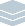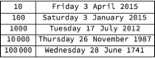Wolfram Archive
Wolfram Programming Lab is a legacy product.
All the same functionality and features, including access to Programming Lab Explorations, are available with Wolfram|One.
Start programming now. »
Try it now »
(no sign-in required)# Powers of Ten History

Find when it was 10, 100, 1,000, 10,000, etc. days ago.

Run the code to find the current date:

This gives todays date:

Today

You can also get yesterdays date and tomorrows:

Yesterday
Tomorrow

Today

Find the date exactly 100 days ago. Try other numbers of days:

DatePlus adds a number of days to a date.

This gives the date 10 days from now:

DatePlus[Today, 10]

This gives the date 100 days ago:

DatePlus[Today, -100]

DatePlus[Today, -100]

Make a table of the first 9 powers of 10. Try different numbers of powers:

Were going to want to look at dates in powers of 10.

This is how to use Table to make a list of the first 9 powers of 10:

Table[10^n, {n, 9}]

Table[10^n, {n, 9}]

Make a table of powers of 10 dates. Try more than 5 dates:

This makes a list of dates in increasing powers of 10 days ago:

Table[DatePlus[Today, -10^n], {n, 5}]

Use DateString to format the date objects as text strings.

This gives today as a date object:

Today

This converts the date object to a text string:

DateString[Today, "Date"]

Make every element of the date list a text string:

Table[DateString[DatePlus[Today, -10^n], "Date"], {n, 5}]

Format the list as a column (you can use either the ...//Column form or the Column[...] form; they mean the same thing):

Table[DateString[DatePlus[Today, -10^n], "Date"], {n, 5}] // Column

Table[DateString[DatePlus[Today, -10^n], "Date"], {n, 5}] // Column

Include the number of days in a table. Try ranges other than 5:

Note: if you change the increment from 10, you have to change both occurrences of 10.

This makes a list of lists. Each sublist has a number of days, and the date that many days ago:

Table[{10^n, DateString[DatePlus[Today, -10^n], "Date"]}, {n, 5}]

Format the list of lists as a grid. Each sublist is a row in the grid:

Grid[Table[{10^n, DateString[DatePlus[Today, -10^n], "Date"]}, {n, 5}]]

Use FrameAll to add frame lines:

Grid[Table[{10^n, DateString[DatePlus[Today, -10^n], "Date"]}, {n, 5}], Frame -> All]

Grid[Table[{10^n, DateString[DatePlus[Today, -10^n], "Date"]}, {n, 5}], Frame -> All]

Share ItMake a website that gives powers of ten tables for dates:

Deploy a form that gives you a power of ten table for a date you specify:

CloudDeploy[FormFunction[{"date" -> "Date"}, Grid[Table[{10^n, DateString[DatePlus[#date, -10^n], "Date"]}, {n, 5}], Frame -> All] &, "PNG"], "Permissions" -> "Public" ]

Click on the link in the output to visit the site.